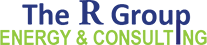## 855.700.7771

This is your own personal Cheat Sheet for calculating demand (kW)

 Key kW = Kilowatt (Demand) kWh = Koliwatt Hours (Energy) I = Current I1 = Phase 1 Current I2 = Phase 2 Current I3 = Phase 3 Current V = Voltage W = Wattage pn = Phase to Neutral pp = Phase to Phase Basic kW Calculation The most basic calculation for demand kWh ÷ Hours = kW Calculating Demand from Pulses If you are trying to calculate Instantaneous Demand and have a pulse meter, try this equation kWh / Pulse x 3,6000 sec/hour —————————————— = kW # of seconds between pulses Single-Phase Calculation Most Single-Phase applications will be for Residential and Small Commercial buildings I x V = W W ÷ 1,000 = kW Balanced 3-Phase Power Measurement Use if all 3 Phases pull the same amount of current I x Vpp x 1.73 = W W ÷ 1,000 = kW Individual 3-Phase Current Measurement Phase to Neutral, Use if the Phases pull different amounts of current. Most simple calculation for these conditions (I1 + I2 + I3) x Vpn = W W ÷ 1,000 = kW Individual 3-Phase Current Measurement Phase to Phase, Another method of calculating Demand if the Phases pull different amounts of current (I1 + I2 + I3) x Vpp x .577 = W W ÷ 1,000 = kW